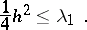# Buser isoperimetric inequality

For a compact Riemannian manifold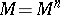, letbe the smallest positive eigenvalue of the Laplace–Beltrami operator (cf. also Laplace–Beltrami equation) ofand define the isoperimetric constant ofby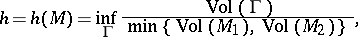wherevaries over the compact hypersurfaces ofwhich partitioninto two disjoint submanifolds,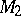.

If the Ricci curvature ofis bounded from below,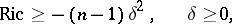then the first eigenvalue has the upper bound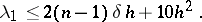Note that a lower bound for the first eigenvalue, without any curvature assumptions, is given by the Cheeger inequality